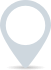# Zero-sum game

A zero-sum game is a situation in game theory and economics where one participant’s gain or loss is exactly balanced by the losses or gains of other participants. In other words, the total amount of wealth, resources, or utility remains constant, and any gain by one participant is offset by an equal loss by another participant. Here are some key points about zero-sum games:

1. Constant total value:
– In a zero-sum game, the total value or wealth in the system remains the same before and after the interactions among participants. This means that any gain by one participant is matched by an equivalent loss by another.

2. Competitive nature:
– Zero-sum games are typically characterised by competition, where one participant’s success directly comes at the expense of another participant’s failure.

3. Examples:
– Some classic examples of zero-sum games include poker (where the total amount of money in play remains constant) and sports competitions (where one team’s victory corresponds to another team’s loss).

4. Zero-sum vs. non-zero-sum:
– Contrastingly, in non-zero-sum games, it is possible for all participants to gain or lose collectively. Cooperative activities, trade, and negotiations often fall into this category.

5. Strict vs. non-strict zero-sum:
– In a strict zero-sum game, the total gains and losses always add up to zero. In a non-strict zero-sum game, there can be slight variations due to factors like transaction costs or information asymmetry.

6. Application in economics:
– In economics, the concept of a zero-sum game is used to analyse situations where resources are distributed or redistributed among participants. It is important in understanding issues like wealth distribution, taxation, and economic policies.

7. Implications for strategy:
– In a zero-sum context, participants are more likely to adopt competitive and aggressive strategies to secure their share of the total value. This can lead to a “win-lose” mentality.

8. Cooperative vs. competitive scenarios:
– While some situations can be modelled as zero-sum games, in reality, many interactions are more complex and can involve elements of cooperation, mutual benefit, and positive-sum outcomes.

9. Limitations:
– It’s important to note that not all real-world situations can be accurately described as zero-sum games. Many economic and social interactions involve complex dynamics where outcomes are not strictly tied to winners and losers.

10. Game theory:
– Zero-sum games are a fundamental concept in game theory, a field of study that analyses interactions among rational decision-makers.

Understanding whether a situation is zero-sum or not is crucial in making informed decisions, especially in competitive environments. While the concept is valuable for theoretical analysis, it’s important to remember that many real-world scenarios involve a mix of competitive and cooperative elements, making them more nuanced than strict zero-sum games.### Clever finance tips and the latest news

#### delivered to your inbox, every week